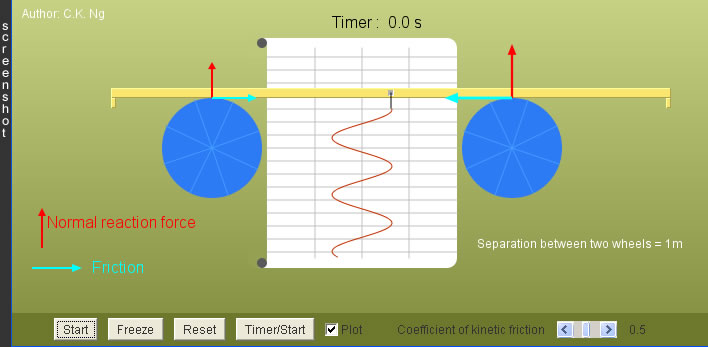SHM Oscillator

1. Drag the beam to one side and then press "Start". Use the timer to record the time for, say, 20 complete cycles.

2. Repeat the above step, but with a larger or smaller amplitude. Is the period independent of amplitude ?

 Definition of SHM: The oscillating motion of an object about a fixed point, such that the acceleration of the object is always directed towards the fixed point, and proportional to the distance from the fixed point. (i) Period is independent of amplitude (isochronism). (ii)Displacement-time graph is sinusoidal.

Question :

A common notion about shm is that kinetic energy is converted to potential energy, and vice versa as the oscillation continues; the sum of the kinetic and potential energy is a constant. In this example, there seems no involvement of any potential energy; where does the kinetic energy come from and go to ?

 Internal Links : Spring-Mass Oscillator (Energy Changes) Pendulum / Interrupted Pendulum Phase Difference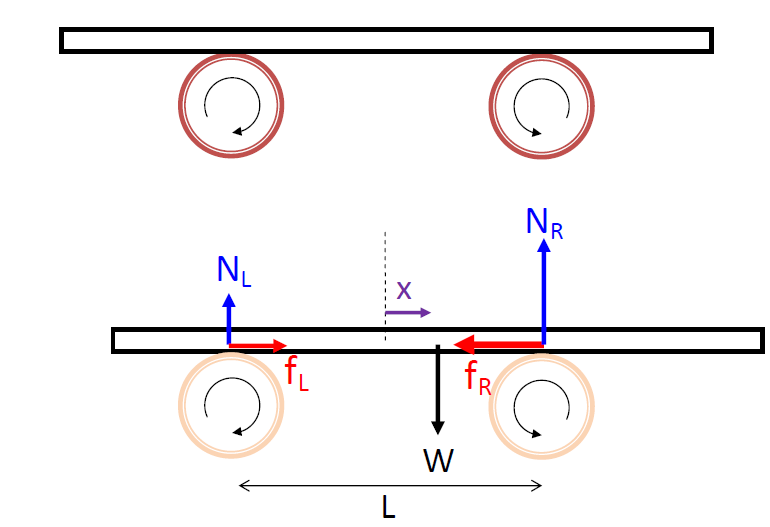A uniform plank of mass m is supported horizontally and symmetrically by two identical rollers of fixed axes and of centers separated by a distance L. The rollers rotate in the opposite directions, always pushing the plank back to the central position, as shown in the above diagrams. Now, the plank is displaced to the right by x. The normal force at the left roller and that at the right, denoted by NL and NR respectively, become unequal. They are (found by the equilibrium of moments)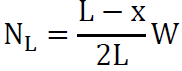and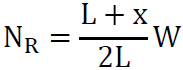, where W = mg is the weight of the plank. Assume the rollers rotate at a fast speed, so the plank always slides on each of them. The frictional forces by the rollers are kinetic, of magnitudes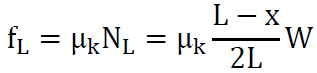and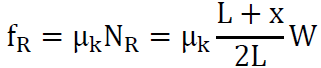, where μk is the coefficient of kinetic friction. Relative to the displacement x, fL is positive and fR is negative. The net force is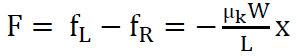. Since a = F/m, we get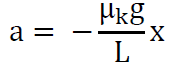. The number μkW/L is positive, so a ∝ –x, satisfying the shm equation a = -ω2x. Finally, we conclude the plank's motion is simple harmonic with angular frequency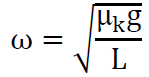.

 The author (Chiu-king Ng) has the copyright on all the simulations in this website. Email phyAA@phy.hk, where AA is the prime number following 7. Last Update:2019-3-20